### Home > A2C > Chapter Ch11 > Lesson 11.1.2 > Problem11-28

11-28.
1. A bag contains eight blue marbles and four gold marbles. If you choose three marbles without looking, what is the probability of getting: Homework Help ✎

1. All blue marbles?

2. Two blue and one gold?

3. All gold marbles?

4. One blue and two gold marbles?

5. Show two different ways to calculate the answer to part (d).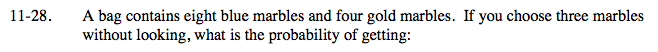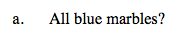$\frac{_8C_3 \cdot _4C_0}{_{12}C_3 }=\frac{14}{55}$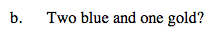$\frac{_8C_2 \cdot _4C_1}{_{12}C_3 }=\frac{28}{55}$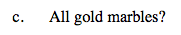See parts (a) and (b).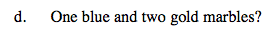See parts (a) and (b).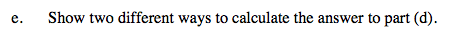How can you solve part (d) using combinations? How can you solve part (d) using the answers to parts (a) through (c)?